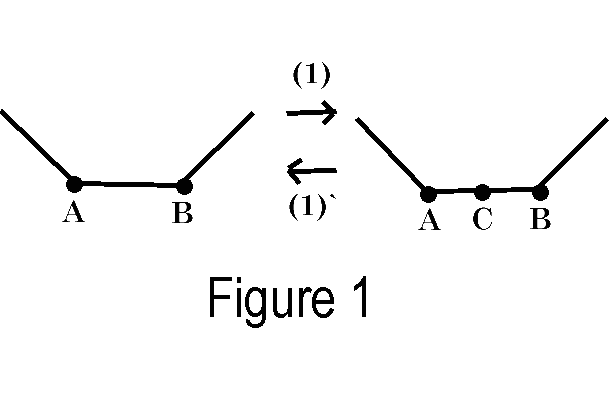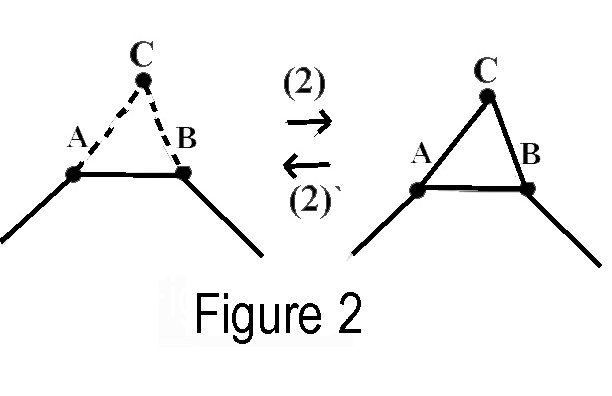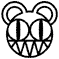# DEFINITION OF A KNOT

Murasugi states the following definition:
On a given knot K we may perform the following four operations, each being applied a finite number of times, to obtain an equivalent knot L:1We may divide an edge AB, in the knot K, into two edges, AC, CB, by placing a point C on the edge AB. (see figure 1)

1` (converse of 1) If AC and CB are two adjacent edges of K such that if C is erased AB becomes a straight line, then we may remove the point C. (see figure 1)2Suppose C is a point in space that does not lie on K.  If the triangle ABC, formed by AB and C, does not intersect K, with the exception of the edge AB, then we may remove AB and add the two edges AC and CB.  (see figure 2)

2` (converse of 2) If there exists in space a triangle ABC that contains two adjacent edges AC and CB of K, and this triangle does not intersect K, except ant the edges AC and CB, then we may delete the two edges AC, CB and add the edge AB. (see figure 2) 

The moves are called the four elementary knot moves.  However, sometimes it is difficult to prove equivalence just by applying the knot moves in a trial-and-error way.  Soon we will see another method of proving equivalence, using something called invariants.kMain menu      1. History              2.Intro               3.Invariants     4.Composition    5.Conclusion